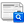Wavelet discrete transform, ANFIS and linear regression for short-term time series prediction of air temperature

(1) * Devi Munandar(Research Center for Informatics – Indonesian Institute of Sciences, Indonesia)
*corresponding authorAbstract

This paper investigates the ability of Discrete Wavelet Transform and Adaptive Network-Based Fuzzy Inference System in time-series data modeling of weather parameters. Plotting predicted data results on Linear Regression is used as the baseline of the statistical model. Data were tested in every 10 minutes interval on weather station of Bungus port in Padang, Indonesia. Mean absolute errors (MAE), the coefficient of determination (R2), Pearson correlation coefficient (r) and root mean squared error (RMSE) are used as performance indicators. The result of Plotting ANFIS data against linear regression using 1-input data is the optimal values combination of output predictions.

Keywords

Discrete Wavelet Transform; Linear Regression; ANFIS; Sub-Time Series Data; Plotting data

DOI

https://doi.org/10.26555/ijain.v3i2.101

Article metrics

Abstract views : 1188 | PDF views : 226

CiteHow to cite item

Full TextDownload

References

B. S. Karthika and P. C. Deka, “Prediction of air temperature by hybridized model (Wavelet-ANFIS) using wavelet decomposed data,” Aquat. Procedia, vol. 4, pp. 1155–1161, 2015.

S. Papantoniou and D.-D. Kolokotsa, “Prediction of outdoor air temperature using neural networks: Application in 4 European cities,” Energy Build., vol. 114, pp. 72–79, 2016.

E. Brulebois, T. Castel, Y. Richard, C. Chateau-Smith, and P. Amiotte-Suchet, “Hydrological response to an abrupt shift in surface air temperature over France in 1987/88,” J. Hydrol., vol. 531, pp. 892–901, 2015.

O. Kisi and M. Cimen, “A wavelet-support vector machine conjunction model for monthly streamflow forecasting,” J. Hydrol., vol. 399, no. 1, pp. 132–140, 2011.

K.-C. Hsu and S.-T. Li, “Clustering spatial--temporal precipitation data using wavelet transform and self-organizing map neural network,” Adv. Water Resour., vol. 33, no. 2, pp. 190–200, 2010.

S. J. Yao, Y. H. Song, L. Z. Zhang, and X. Y. Cheng, “Wavelet transform and neural networks for short-term electrical load forecasting,” Energy Convers. Manag., vol. 41, no. 18, pp. 1975–1988, 2000.

X. An, D. Jiang, C. Liu, and M. Zhao, “Wind farm power prediction based on wavelet decomposition and chaotic time series,” Expert Syst. Appl., vol. 38, no. 9, pp. 11280–11285, 2011.

K. Wilgan, W. Rohm, and J. Bosy, “Multi-observation meteorological and GNSS data comparison with Numerical Weather Prediction model,” Atmos. Res., vol. 156, pp. 29–42, 2015.

G. A. Fallah-Ghalhary, M. Mousavi-Baygi, and M. H. Nokhandan, “Annual Rainfall Forecasting by Using Mamdani Fuzzy Inference System,” Res. J. Environ. Sci., vol. 3, no. 4, pp. 400–413, 2009.

N. Anwer, A. Abbas, A. Mazhar, and S. Hassan, “Measuring weather prediction accuracy using sugeno based Adaptive Neuro Fuzzy Inference system, grid partitioniong and guassmf,” in Computing Technology and Information Management (ICCM), 2012 8th International Conference on, 2012, vol. 1, pp. 214–219.

M. Yousefi, D. Hooshyar, A. Remezani, K. S. M. Sahari, W. Khaksar, and F. B. I. Alnaimi, “Short-term wind speed forecasting by an adaptive network-based fuzzy inference system (ANFIS): an attempt towards an ensemble forecasting method,” Int. J. Adv. Intell. Informatics, vol. 1, no. 3, pp. 140–149, 2015.

D. Munandar, "Optimization weather parameters influencing rainfall prediction using Adaptive Network-Based Fuzzy Inference Systems (ANFIS) and linier regression," 2015 International Conference on Data and Software Engineering (ICoDSE), Yogyakarta, 2015, pp. 1-6International Journal of Advances in Intelligent InformaticsThis work is licensed under a Creative Commons Attribution-ShareAlike 4.0